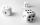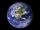Cube from sphere

What largest surface area (in cm2) can have a cube that was cut out of a sphere with radius 43 cm?

Result

A =  14792 cm2

Solution:Leave us a comment of example and its solution (i.e. if it is still somewhat unclear...):

Showing 1 comment:Math student
Nice puzzle. It took me a moment to realise my mistake and use 3D Pythagoras so I guess a hint might be helpful to others.To solve this example are needed these knowledge from mathematics:

Pythagorean theorem is the base for the right triangle calculator.

Next similar examples:

1. Cube surface areaThe surface of the cube was originally 216 centimeters square. The surface of the cube has shrunk from 216 to 54 centimeters sq. Calculate how much percent the edge of the cube has decreased.
2. Sphere vs cubeHow many % of the surface of a sphere of radius 12 cm is the surface of a cube inscribed in this sphere?
3. Three diceWhen you throw three dice was the sum total of the dice 10. The yellow dice fell one eye more than on the red and brown fell 3 eyes less than red. How many eyes fell on every dice?
4. Theorem proveWe want to prove the sentence: If the natural number n is divisible by six, then n is divisible by three. From what assumption we started?
5. Fit ballWhat is the size of the surface of Gymball (FIT - ball) with a diameter of 65 cm?
6. Earth's surfaceThe greater part of the earth's surface (r = 6371 km) is covered by oceans; their area is approximately 71% of the Earth's surface. What is the approximate area of the land?
7. First manWhat is the likelihood of a random event where are five men and seven women first will leave the man?
8. Linear systemSolve a set of two equations of two unknowns: 1.5x+1.2y=0.6 0.8x-0.2y=2
9. Functions f,gFind g(1) if g(x) = 3x - x2 Find f(5) if f(x) = x + 1/2
10. 6 termsFind the first six terms of the sequence. a1 = 7, an = an-1 + 6
11. Nineteenth memberFind the nineteenth member of the arithmetic sequence: a1=33 d=5 find a19
12. Factory and divisionsThe factory consists of three auxiliary divisions total 2,406 employees. The second division has 76 employees less than 1st division and 3rd division has 212 employees more than the 2nd. How many employees has each division?
13. Volleyball8 girls wants to play volleyball against boys. On the field at one time can be six players per team. How many initial teams of this girls may trainer to choose?
14. PowersExpress the expression ? as the n-th power of the base 10.
15. Two equationsSolve equations (use adding and subtracting of linear equations): -4x+11y=5 6x-11y=-5
16. TetrahedronCalculate height and volume of a regular tetrahedron whose edge has a length 18 cm.
17. TreesA certain species of tree grows an average of 0.5 cm per week. Write an equation for the sequence that represents the weekly height of this tree in centimeters if the measurements begin when the tree is 200 centimeters tall.